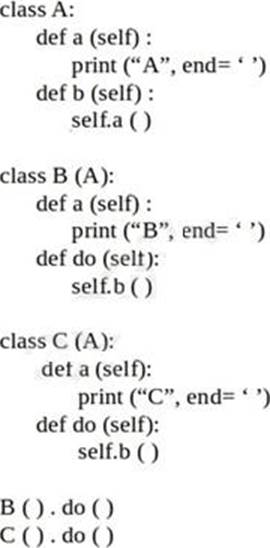Take a free PCAP-31-03 practice test to help you prepare for the exam.

Page 1 of 4

1. What is the expected behavior of the following code?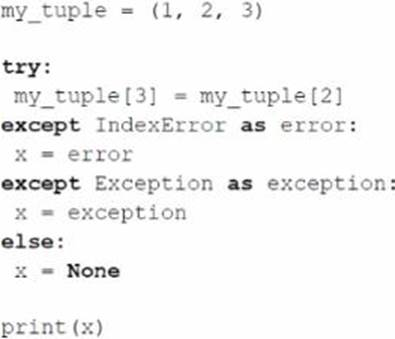2. Assuming that the following piece of code has been executed successfully, which of the expressions evaluate to True? (Select two answers)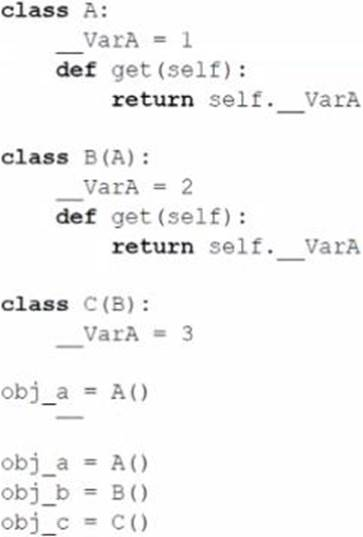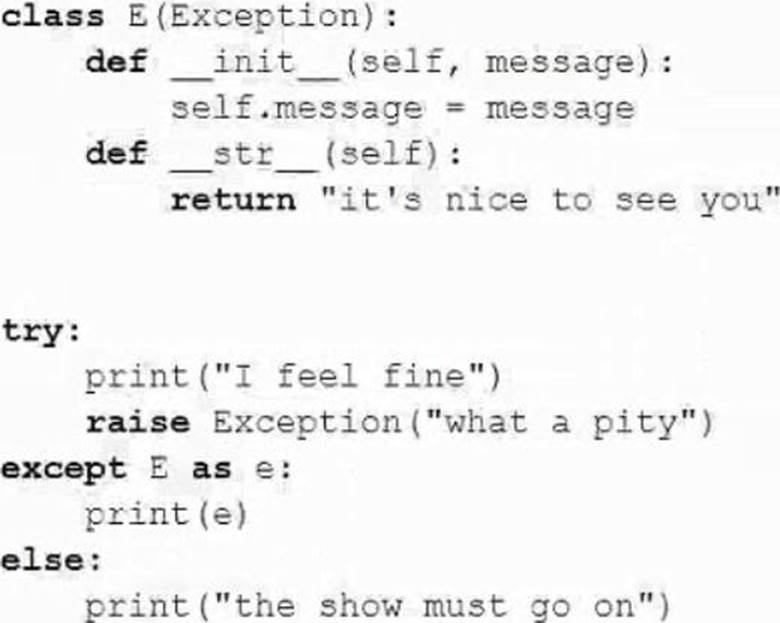4. Which of the following lines of code will work flawlessly when put independently inside the inc ( ) method in order to make the snippet’s output equal to 3? (Select two answers)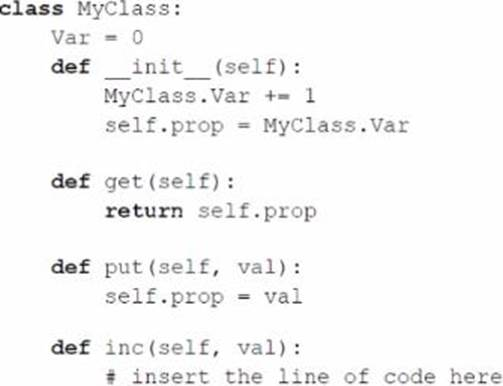5. What is the expected behavior of the following code?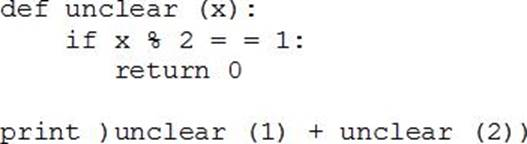It will:

6. What is the expected behavior of the following code?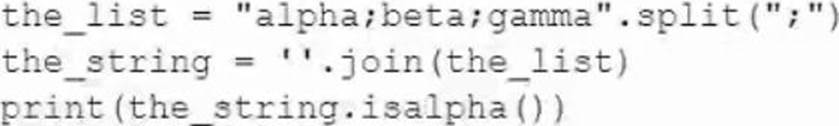7. Assuming that the following code has been executed successfully, selected the expression which evaluate to True (Select two answers)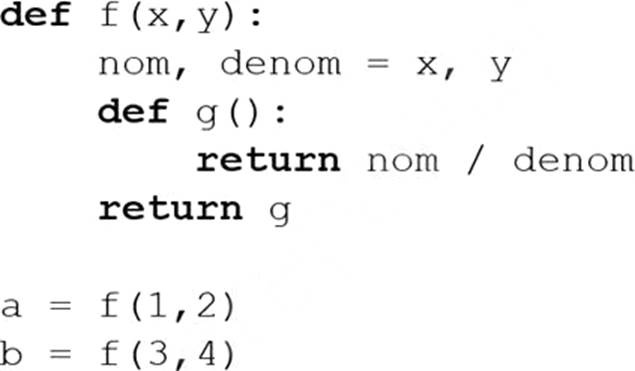8. The following class hierarchy is given .

What is the expected output of the code?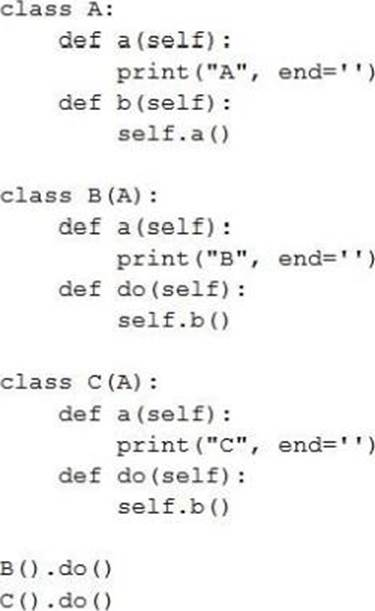9. The following class hierarchy is given .

What is the expected out of the code?